# Asg 6: photon mapper

## Objectives

To implement a photon mapper: store collected photons in a kd-tree, then run your ray tracer to accumulate and render caustics

## Assignment

1. Once photons have been shot into the scene and they are stored in a `std::vector` of pointers to photons, the next step is to scale each of the "stuck" photons' power by 1/n where n is the number of stuck photons—note that it may not be the same number as the number of photons shot out as some of these did not stick.
(This can be done in `main()`.)
2. After photon power normalization, find the smallest and largest photons in terms of their positions, and then use these as the min and max bounds to insert the `std::vector` of pointers to photons into your kd-tree.
(This can be done in `main()`.)
3. Once the kd-tree has been created, then the `ray_t::trace()` routine is augmented such that it accepts a reference to the kd-tree as an additional argument, and then as a final step in the routine, the k nearest photons are collected at each ray's hit point, and an estimate or the radiance at that point is calculated—this is very similar to calculating the specular reflection at the hit point. The key observation here is that the radiance estimate depends on the density of photons at the hit point—if there are a lot of tightly packed photons (e.g., as you would expect at the location of a caustic), then the radius r returned by the k-nearest neighbor kd-tree query will be smaller than at hit points where photon density is smaller. (This can be done in `ray_t::trace()`.)
4. For manageable render times, shoot 2,000 photons into the scene, and each hit point, sample only 20 photons.
(This can be done in `model_t::shoot()`.)
5. Because the kd-tree knn query accepts as arguments a photon, the knearest std::vector<photon_t* > array of nearest photons, r, and k, "masquerade" the hit point as a photon by temporarily constructing a photon with the hit point as its position, e.g., ``` photon_t *query = new photon_t(hit,vec_t(0.0,0.0,0.0),vec_t(0.0,0.0,0.0)); ``` then call the kd-tree knn query: ``` kdtree.knn(query,knearest,radius,20); ``` which will return an array of k nearest photons and r, the distance the kth one. The idea is to calculate all the radiance flux from all gathered photons.
(This can be done in `ray_t::trace()`.)
6. To compute flux, for each of the k nearest photons:
• check the angle between the surface normal N and the negated photon direction -u·N, if this is > 0 then use the photon in the computation of flux, otherwise ignore it
• flux is simply a sum of the power of all photons with -u·N > 0
• flux can be represented as a `rgb_t<double>` type, just as was done previously for reflected or transmitted color or specular or diffuse contributions
(This can be done in `ray_t::trace()`.)
7. Once the flux has been computed, scale it by 1/(πr2) and add it to the resultant color, remembering to clamp the color to (0.0,1.0) as with all the other color contributions. (This can be done in `ray_t::trace()`.)

## Results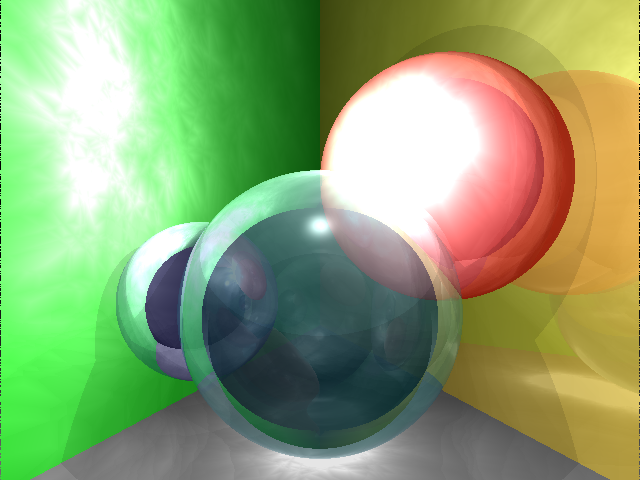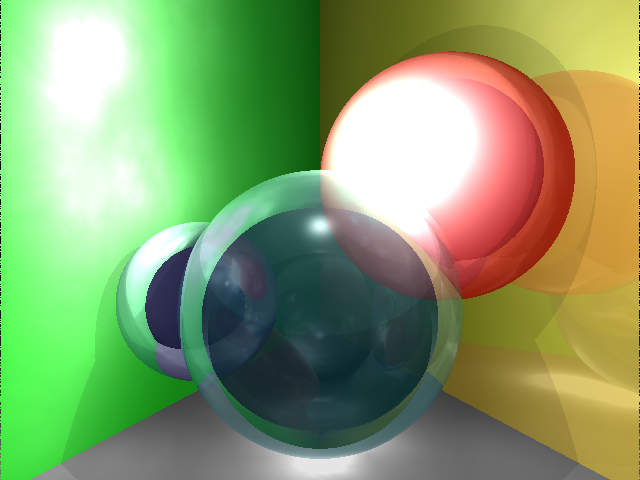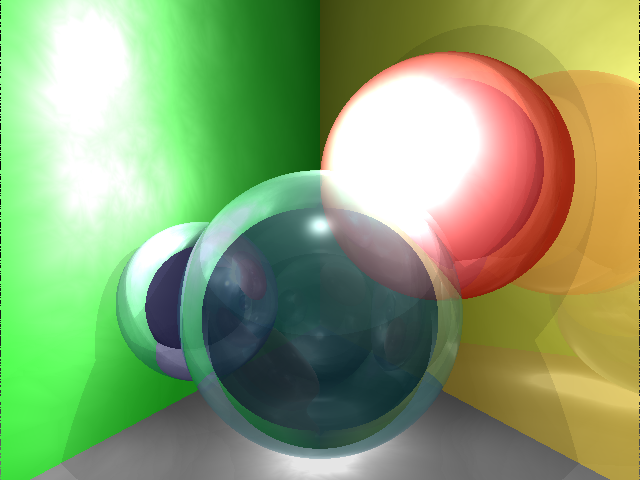air sphere, one light 2,000 photons per light, 20 photons sampled 10 ray bounces, 10 photon bounces 8 cores: 85.3 s, 8 cores: 56.3 s air sphere, one light, cone filter 2,000 photons per light, 20 photons sampled 10 ray bounces, 10 photon bounces 8 cores: 84.8 s air sphere, one light, Gaussian filter 2,000 photons per light, 20 photons sampled 10 ray bounces, 10 photon bounces 8 cores: 84.8 s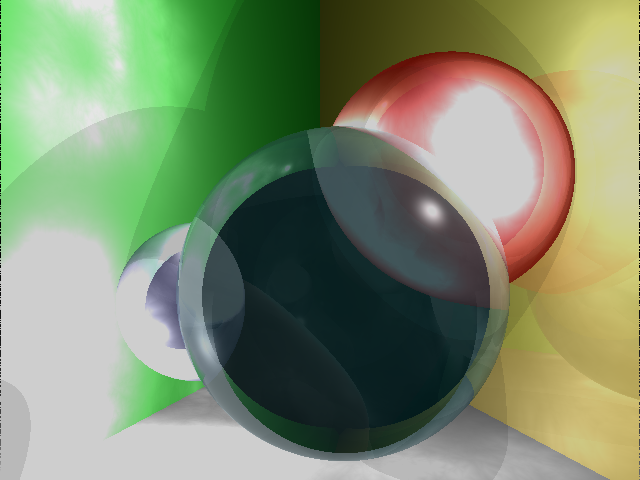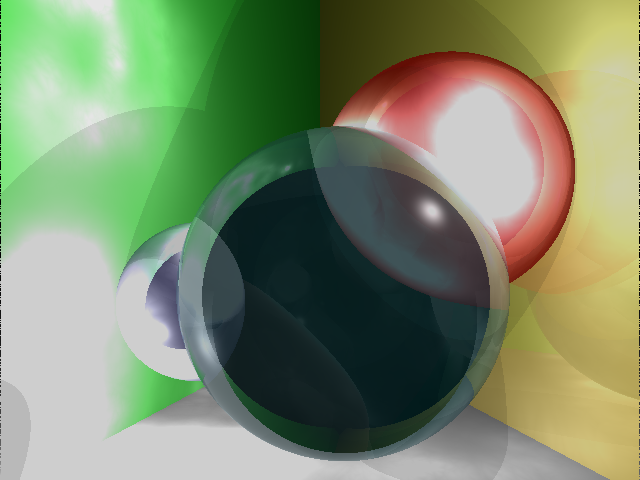final scene 2,000 photons per light, 20 photons sampled 10 ray bounces, 10 photon bounces 8 cores: 107.1 s, 8 cores: 81.4 s final scene, cone filter 2,000 photons per light, 20 photons sampled 10 ray bounces, 10 photon bounces 8 cores: 107.2.7 s final scene, Gaussian filter 2,000 photons per light, 20 photons sampled 10 ray bounces, 10 photon bounces 8 cores: 106.3 s

## Suggestions

1. Three `photon_t` class public member functions/operators are very important to get everything working properly:
• `operator<` and `operator>`: these should provide order information in terms of photon position—that is, if all of the x, y, z coordinates of `this` photon are smaller than the `rhs`, then `this` photon is "smaller" than `rhs`
• `operator[]` makes things a bit cleaner when it is made to return the ith coordinate, i.e., `(*this)` returns x
• `double distance(const vec_t& rhs)` and `double distance(const photon_t& rhs)`: overloaded functions that compute the Euclidean distance between `this` photon and `rhs` if `rhs` is a photon ``` vec_t diff = pos - rhs.pos; return(sqrt(diff.dot(diff))); ``` or ``` vec_t diff = pos - rhs; return(sqrt(diff.dot(diff))); ``` or if `rhs` is a `vec_t` (assuming your `vec_t` class has both `operator-` and `dot()` functionality)
2. For the kd-tree to work properly, besides the above, your `photon_t` interface should also define a `photon_c` functor, or function object which has as its only public member function the overloaded `bool operator()` operator that returns `true` when two `photon_t` objects are compared with `operator<`, e.g., ``` bool operator()(const photon_t& p1, const photon_t& p2) const { return(p1[axis] < p2[axis]); } ``` where `axis` is `photon_c`'s `private` integer data member that is optionally initialized to 0 upon construction: ``` public: photon_c(int inaxis=0) : axis(inaxis) {}; private: int axis; ``` so that the kd-tree can use this funcor to order photons during insertion and queries.
3. For debugging purposes, you should edit the model file you're using to reduce the size of the image you are trying to generate: for "quick-and-dirty" debugging, use something very small like 64 x 48 to see if you're getting any kind of caustic effect at all...if it looks right, try 320 x 240 before going on to the our "full-size" 640 x 480 image.

## Optional

1. Once you have all of the above working and are able to produce unfiltered images as above, try either of the cone or Gaussian filters to smooth the somewhat cloudy appearance of the radiance estimate:
• the cone filter is calcuated as
```		wpc = (1.0 - (dp/(k * r)))/(1.0 - (2.0/(3.0*k)));
```
where dp is the distance from the hit point to the current photon, and k = 1.1 (this is just a constant, not to be confused with the k in the k nearest neighbor idea (where k = 20)
• the Gaussian filter is calculated as
```		wpg = α * ( 1.0 - ((1.0 - exp(-β*dp2/(2.0*r2))) / (1.0 - exp(-β))) );
```
with α = 1.818 and β = 1.953
and each of wpc and wpg are just used to scale the power of each of the photons that is used in the flux computation

## Turn in

Turn in all of your code, in one `tar.gz` archive of your asg##/ directory, including:
1. A `README` file containing
1. Course id--section no
2. Name
3. Lab description
4. Brief solution description (e.g., program design, description of algorithm, etc., however appropriate).
5. Lessons learned, identified interesting features of your program
6. Any special usage instructions
2. `Makefile`
3. source code (`.h` headers and `.cpp` source)
4. object code (do a `make clean` before `tar`)

## How to hand in

See `handin` notes